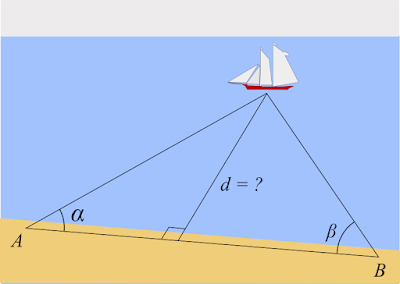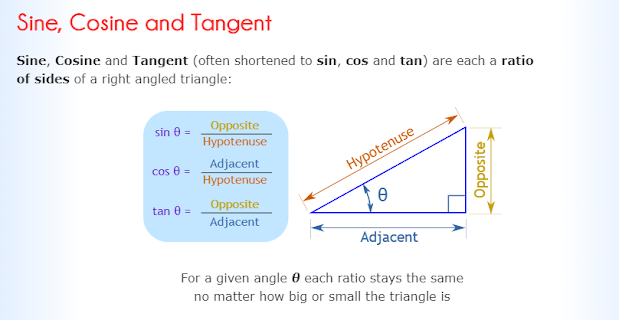## Friday, November 25, 2022

### Finding the AngleNot actually Erin.Picture by KJ Photography& is used with permission.
Special thanks to George Groot for double-checking my math and helping with clarity.

I realized that my previous post referenced using azimuths to find an angle without explicitly showing how to do that. I will attempt to explain the process, but please understand that math is not my best subject, and I may flail verbally about while trying to make my point.

Triangulation is a process used in surveying and navigation which uses triangles (hence the name) to determine the coordinates of a point. You need two known angles and one known side length (or distance) to do this, creating a triangle which allows us to use trigonometry to solve for the other angles and distances.https://en.wikipedia.org/wiki/Triangulation_(surveying)

Let us assume that you need to find the distance to the boat in the illustration above. How do you do that without a rangefinder?

1) Starting at point A, shoot an azimuth to the boat and record it.

2) Using your compass, you turn right 90° and march toward point B. I recommend 10 yards, as it is a short enough distance that your bearing will not vary too much, and it is a nice round number that ought to make math easier.
How will I know when I've traveled 10 yards? If you're doing land navigation, you should know your pace count per 100 yards, so just divide that by 10 and when you've walked that number of paces, you have arrived. If you don't know your pace count, this article by Lokidude will show you how.
If the object is quite large, you might need to take more steps; I still suggest you take them in units of 10.
I am making this slightly more complicated than it strictly needs to be, because you will need to have a straight line (d) coming from the boat that intersects line AB at a right angle. If you don't have a right angle, things will be much more complicated and outside the scope of this article, so I'm having you do a little more work now to save you a lot more grief later.

This is the same reason I'm not having you walk until you're at 90° to the boat. What is the likelihood that you'll correctly make a 90° angle the first time? To my mind, this extra step prevents that mistake.

3) At point B, shoot a second azimuth to the boat and record it.

4) Shoot a third azimuth to point A and record that.

5) In a triangle, no single angle can be more than 90°, and we're going to create a 90° angle in a moment, so angle ß cannot be larger than 90°. If it is, then subtract it from 180°. If it isn't, then subtract it from 90°.

6) You now know angle ß. Record it. (For this example we will say it is 60°.)

7) Halve the distance of line AB to create two right-angle triangles which share a side, d. Side d is the distance you need to determine.

8) You know the distance from B to D (the halfway point between A and B) is 5 yards. You know the measurement of angle ß. This is all you need, so now let's do some math.

9) How much do you remember from Geometry?https://www.mathsisfun.com/sine-cosine-tangent.html

We need to find the length of d, which in this figure is the Opposite side. The tangent of angle ß is the quotient (the number you get as a result of division) of the Opposite over the Adjacent.
Side note: if you ever forget, just remember SOH CAH TOA:
• Sine is the Opposite over the Hypotenuse (SOH)
• Cosine is the Adjacent over the Hypotenuse (CAH)
• Tangent is the Opposite over the Adjacent (TOA)
But we don't need to find that angle, because we know it. So we do some math jiggery-pokery:
• Tangent of Θ = O/A
• Multiply both sides by A
• A times tangent Θ = O
Which is exactly the same formula which David's friend gave us here, just with different names for the sides.

So going back to the original problem with sailboat distance:

We know the distance from B to D is 5 yards.

We know the measurement of angle ß is 60°.

Therefore, 5 times the tangent of 60° will give us the length of line d, the distance to our target.

The tangent of 60° is 1.73205080757. Shortening that to 1.73 ought to suffice.

Therefore, d = 5 * 1.73.

Therefore d -- the straight line distance from shore to boat -- is 8.65 yards.

The Right Angle Theorem says that the length of the side opposite the right angle is the square of the sum of the squares of the other two sides. In other words, the famous A² + B² = C², where C is the side opposite the right angle, also known as the Hypotenuse.

Therefore, 5² + 8.65² = C².

Therefore, 25 + 74.8225 = C².

Therefore, C² = 99.8225.

Take the square root of both sides.

C = 9.9911 yards.

So the distance from your current position at point B to the sailboat is almost 10 yards, or 30 feet.

This makes good intuitive sense, as solving for all angles shows that we originally had a 60/60/60 equilateral triangle, in which case the distance between A and Boat and B and Boat must be the same as the distance between A and B.
Remember, we know that we have a 90° angle and a 60° angle, and all angles within a triangle must add up to 180°, so 90 + 60 = 150 and 180 - 150 = 30, therefore the remaining angle opposite side DB must be 30°.

Since this triangle is half of our original one, the other half must be symmetrical and therefore identical. Therefore, the angle opposite side AB is 60°.

We can exploit this feature of angles without using a compass for short distances, such as distance across streams or small rivers, simply by using the bill of a cap to align with the distant shore and  then turning to face a point on land without moving your head. The bill of the cap swung in an arc, and the arc describes the same distance (a radius) from the central point, letting you determine a distance that you can’t walk to by walking to a point on the same arc that you can reach. This is much less math than using triangulation to find the distance, and much quicker to determine things like "Do I have enough rope to make a rope bridge?"

If trigonometric functions are confusing, an alternate way is to use "minute of angle math" to determine the distance. Starting at point A, shoot an azimuth to your unknown distance target. Turn 90 degrees to walk parallel to the target for a known distance and shoot a second azimuth to the target. Subtract the smaller angle from the larger angle to get the "inside angle" of the target to your known distance.

Since one minute of angle is roughly one inch at one hundred yards, if your known distance was 10 feet (120 inches) and your angle was 1 degree (60 minutes) you know that your unknown distance to the target is 200 yards away (60 minutes = 120 inches at 200 yards). This method requires a precise compass, and precise linear measurement, but it does work.